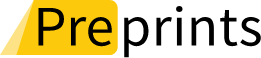## Topic EditorsDepartment of Engineering Sciences, İzmir Katip Çelebi University, İzmir 35620, TurkeyDr. Asif Khan
Department of Mathematics, Aligarh Muslim University, Aligarh 202002, IndiaDr. Syed Abdul Mohiuddine
1. Operator Theory and Applications Research Group, Department of Mathematics, Faculty of Science, King Abdulaziz University, Jeddah 21589, Saudi Arabia
2. Department of General Required Courses, Mathematics, Faculty of Applied Studies, King Abdulaziz University, Jeddah 21589, Saudi ArabiaDepartment of Engineering Sciences, İzmir Katip Çelebi University, İzmir 35620, Turkey

# Approximation Theory in Computer and Computational Sciences

20 June 2023
20 September 2023
Viewed by
1948

## Topic Information

Dear Colleagues,

In recent years, we have observed increasing interest of Mathematicians in Approximation theory and computational methods, due to its large applications in Engineering sciences and related areas. Approximating functions, some data, or a member of a given set are some of the examples of the approximation calculations. From constructive proof of the Weierstrass approximation theorem via Bernstein polynomials to the theory of positive linear operators, role of Bernstein type polynomials to mimic shapes of curves and surfaces in Computer-Aided Geometric Design and its applications to finite element analysis, approximation by non-linear operators, Neural network approximation and the theory of sampling operators to overcome complexity of Mathematical models are some of the key areas. Approximation theory links both theoretical and applied Mathematics from a need to represent functions in computer calculations to Numerical analysis and development of mathematical software etc. Any development can be used in many industrial and commercial fields and thus requires advances in the subject.

In this Topic Issue, we will cover the field of approximations in Neural Networks, Linear and Non-linear approximation operators, Numerical analysis, Special function classes, Computer Aided Geometric design and applications of approximation theory. Our goal is to gather articles reflecting new trends in approximation theory and related topics.

Dr. Faruk Özger
Dr. Asif Khan
Dr. S.A. Mohiuddine
Dr. Zeynep Ödemiş Özger
Topic Editors

## Keywords

• best approximant
• neural network operators
• sigmoidal functions
• pointwise and uniform approximation
• rate of convergence
• sampling operators
• linear and nonlinear approximating operators
• error estimate
• Bernstein type operators
• Bezier type curves and surfaces

## Participating Journals

Journal Name Impact Factor CiteScore Launched Year First Decision (median) APCAlgorithms
algorithms
- 3.3 2008 17.6 Days 1600 CHFAxioms
axioms
1.824 2.6 2012 17.8 Days 1600 CHFFractal and Fractional
fractalfract
3.577 2.8 2017 18.3 Days 1800 CHFMathematics
mathematics
2.592 2.9 2013 16.8 Days 2100 CHFSymmetry
symmetry
2.940 4.3 2009 14.2 Days 2000 CHFPreprints is a platform dedicated to making early versions of research outputs permanently available and citable. MDPI journals allow posting on preprint servers such as Preprints.org prior to publication. For more details about reprints, please visit https://www.preprints.org.

## Published Papers (2 papers)

Order results
Result details
Journals
Select all
Export citation of selected articles as:
Article
Influence of Spatial Dispersal among Species in a Prey–Predator Model with Miniature Predator Groups
Symmetry 2023, 15(5), 986; https://doi.org/10.3390/sym15050986 - 26 Apr 2023
Viewed by 512
Abstract
Dispersal among species is an important factor that can govern the prey–predator model’s dynamics and cause a variety of spatial structures on a geographical scale. These structures form when passive diffusion interacts with the reaction part of the reaction–diffusion system in such a [...] Read more.
Dispersal among species is an important factor that can govern the prey–predator model’s dynamics and cause a variety of spatial structures on a geographical scale. These structures form when passive diffusion interacts with the reaction part of the reaction–diffusion system in such a way that even if the reaction lacks symmetry-breaking capabilities, diffusion can destabilize the symmetry and allow the system to have them. In this article, we look at how dispersal affects the prey–predator model with a Hassell–Varley-type functional response when predators do not form tight groups. By considering linear stability, the temporal stability of the model and the conditions for Hopf bifurcation at feasible equilibrium are derived. We explored spatial stability in the presence of diffusion and developed the criterion for diffusion-driven instability. Using amplitude equations, we then investigated the selection of Turing patterns around the Turing bifurcation threshold. The examination of the stability of these amplitude equations led to the discovery of numerous Turing patterns. Finally, numerical simulations were performed to validate the outcomes of the analysis. The outcomes of the theoretical study and numerical simulation were accorded. Our findings demonstrate that spatial patterns are sensitive to dispersal and predator death rates. Full article
Show FiguresFigure 1

Article
On the Approximation by Bivariate Szász–Jakimovski–Leviatan-Type Operators of Unbounded Sequences of Positive Numbers
Mathematics 2023, 11(4), 1009; https://doi.org/10.3390/math11041009 - 16 Feb 2023
Viewed by 476
Abstract
In this paper, we construct the bivariate Szász–Jakimovski–Leviatan-type operators in Dunkl form using the unbounded sequences ${\alpha }_{n}$${\beta }_{m}$ and ${\xi }_{m}$ of positive numbers. Then, we obtain the rate of convergence in terms of the weighted modulus of continuity of [...] Read more.
In this paper, we construct the bivariate Szász–Jakimovski–Leviatan-type operators in Dunkl form using the unbounded sequences ${\alpha }_{n}$${\beta }_{m}$ and ${\xi }_{m}$ of positive numbers. Then, we obtain the rate of convergence in terms of the weighted modulus of continuity of two variables and weighted approximation theorems for our operators. Moreover, we provide the degree of convergence with the help of bivariate Lipschitz-maximal functions and obtain the direct theorem. Full article